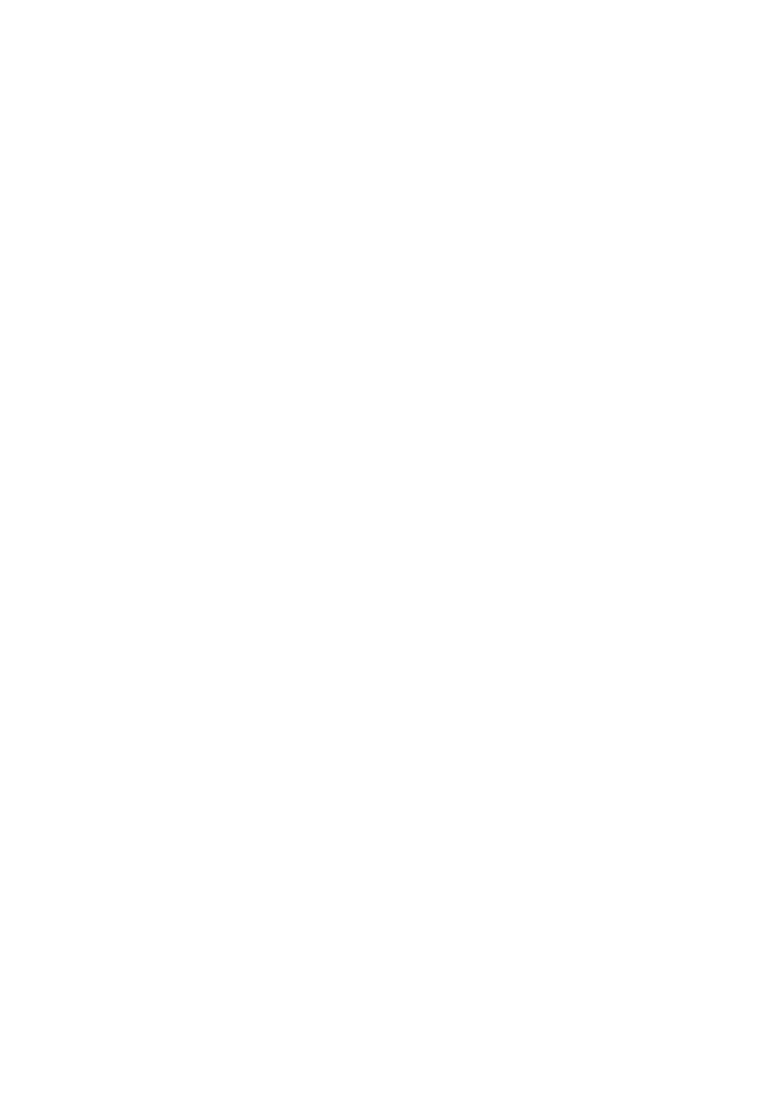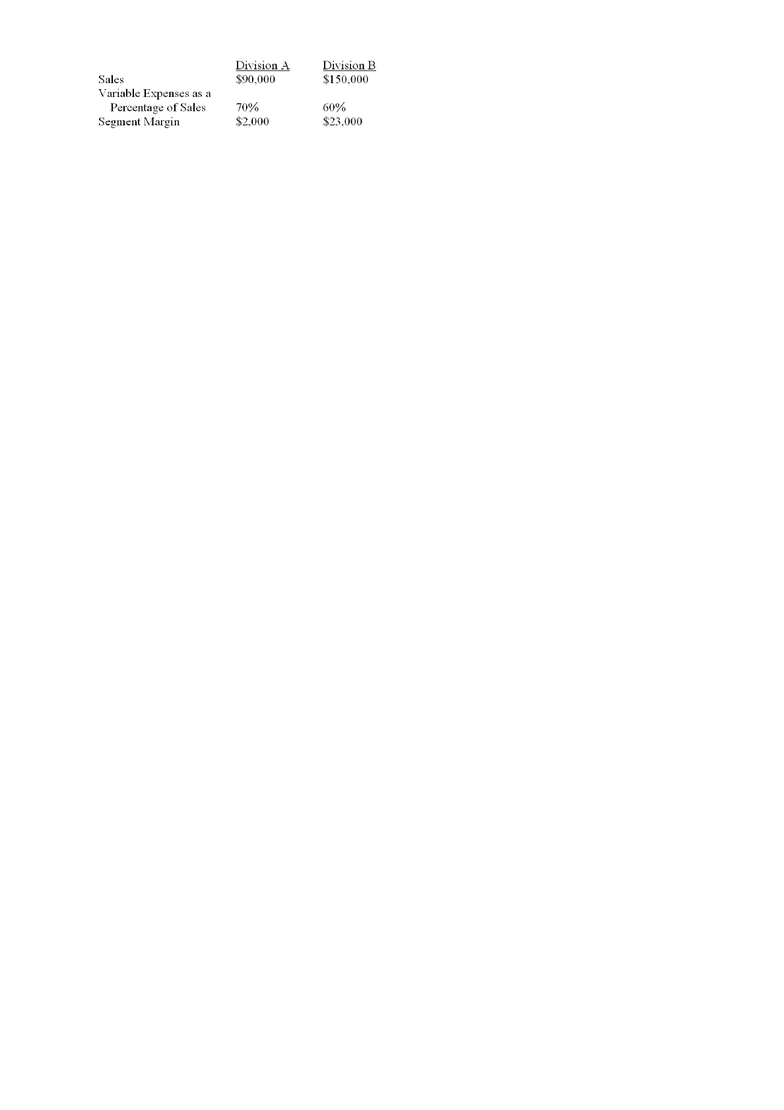Study Guides (390,000)
CA (150,000)
York (10,000)
Final

Department
Course Code
Professor
Alison Beavis
Study Guide
Final

This preview shows pages 1-3. to view the full 57 pages of the document.ch11
Student: ___________________________________________________________________________
1. Which of the following is the numerator in the calculation of the turnover component of ROI?
A. Invested capital.
B. Total assets.
C. Operating income.
D. Sales.
2. What would be a good example of a common cost that normally could NOT be assigned to products on a
segmented income statement except on an arbitrary basis?
B. Salary of a corporation president.
C. Direct materials.
D. The product manager's salary.
3. All other things being equal, which of the following is a consequence of an increase in a division's
traceable fixed expenses?
A. The division's contribution margin ratio will decrease.
B. The division's segment margin ratio will remain the same.
C. The division's segment margin will decrease.
D. The overall company operating income will remain the same.
4. Lyons Company consists of two divisions: A and B. Lyons Company reported a contribution margin of
\$50,000 for Division A, and had a contribution margin ratio of 30% in Division B, when sales in Division
B were \$200,000. Operating income for the company was \$25,000 and traceable fixed expenses were
\$40,000. What were Lyons Company's common fixed expenses?
A. \$40,000.
B. \$45,000.
C. \$70,000.
D. \$85,000.
5. More Company has two divisions: L and M. During July, the contribution margin in Division L was
\$60,000. The contribution margin ratio in Division M was 40%, and its sales were \$250,000. Division
M's segment margin was \$60,000. The common fixed expenses were \$50,000, and the company operating
income was \$20,000. What was the segment margin for Division L?
A. \$0.
B. \$10,000.
C. \$50,000.
D. \$60,000.
6. During April, Division D of Carney Company had a segment margin ratio of 15%, a variable expense
ratio of 60% of sales, and traceable fixed expenses of \$15,000. Division D's sales were closest to which
of the following?
A. \$22,500.
B. \$33,333.
C. \$60,000.
D. \$100,000.

Only pages 1-3 are available for preview. Some parts have been intentionally blurred.7. Divisions A and B of Denner Company reported the following results for October:
If common fixed expenses were \$31,000, what were the total fixed expenses?
A. \$31,000.
B. \$52,000.
C. \$62,000.
D. \$93,000.
8. Johnson Company operates two plants: Plant A and Plant B. Johnson Company reported for the year just
ended a contribution margin of \$50,000 for Plant A. Plant B had sales of \$200,000 and a contribution
margin ratio of 30%. Net operating income for the company was \$20,000 and traceable fixed costs for the
two plants totalled \$50,000. What were Johnson Company's common fixed costs for last year?
A. \$40,000.
B. \$50,000.
C. \$70,000.
D. \$90,000.
Ieso Company has two stores: J and K. During November, Ieso Company reported operating income of
\$30,000 and sales of \$450,000. The contribution margin in Store J was \$100,000, or 40% of sales. The
segment margin in Store K was \$30,000, or 15% of sales. Traceable fixed expenses were \$60,000 in Store
J, and \$40,000 in Store K.
9. What were the total sales in Store J?
A. \$100,000.
B. \$150,000.
C. \$250,000.
D. \$400,000.
10. What were the total variable expenses in Store K?
A. \$70,000.
B. \$110,000.
C. \$130,000.
D. \$200,000.
11. What were Ieso Company's total fixed expenses for the year?
A. \$40,000.
B. \$100,000.
C. \$140,000.
D. \$170,000.
12. What was the segment margin ratio in Store J?
A. 16%.
B. 24%.
C. 40%.
D. 60%.
Canon Company has two sales areas: North and South. During last year, the contribution margin in the
North was \$50,000, or 20% of sales. The segment margin in the South was \$15,000, or 8% of sales.
Traceable fixed costs were \$15,000 in the North and \$10,000 in the South. During last year, the company
reported total operating income of \$26,000.

Only pages 1-3 are available for preview. Some parts have been intentionally blurred.13. What were the total fixed costs (traceable and common) for Canon Company for the year?
A. \$24,000.
B. \$25,000.
C. \$49,000.
D. \$50,000.
14. What were the variable costs for the South area for the year?
A. \$65,000.
B. \$162,500.
C. \$185,000.
D. \$230,000.
15. Which of the following statements provide(s) an argument in favour of including only a plant's net book
value rather than gross book value as part of operating assets in the ROI computation?
I. Net book value is consistent with how plant and equipment items are reported on a balance sheet.
II. Net book value is consistent with the computation of operating income, which includes amortization as
an operating expense.
III. Net book value allows ROI to decrease over time as assets get older.
A. I only.
B. III only.
C. I and II only.
D. I and III only.
16. In computing the margin in a ROI analysis, which of the following is used?
A. Sales in the denominator.
B. Operating income in the denominator.
C. Average operating assets in the denominator.
D. Residual income in the denominator.
17. Which of the following is NOT an operating asset?
A. Cash.
B. Inventory.
C. Plant equipment.
D. Common shares.
18. Assuming that sales and operating income remain the same, which of the following statements about a
company's return on investment is correct?
A. It will increase if operating assets increase.
B. It will decrease if operating assets decrease.
C. It will decrease if turnover decreases.
D. It will decrease if turnover increases.
19. All other things equal, which of the following events would generally cause an increase in a company's
return on investment (ROI)?
A. An increase in average operating assets.
B. A decrease in sales.
C. A decrease in operating expenses.
D. An increase in operating expenses.
20. How is a company's return on investment calculated?
A. Dividing the margin by the turnover.
B. Multiplying the margin by the turnover.
C. Dividing the turnover by the average operating assets.
D. Multiplying the turnover by the average operating assets.